Courses

# Deep Foundations Notes | EduRev

## Civil Engineering (CE) : Deep Foundations Notes | EduRev

The document Deep Foundations Notes | EduRev is a part of the Civil Engineering (CE) Course Foundation Engineering.
All you need of Civil Engineering (CE) at this link: Civil Engineering (CE)

Bearing capacity of piles

The ultimate bearing capacity of a pile is the maximum load which it can carry without failure or excessive settlement of the ground. The bearing capacity also depends on the methods of installation

1. Analytical Method
(i) Qup = Qeb + Qsf
(ii) Qup = qbAb + qsAs
where,
Qup = Ultimate load on pile
Qeb = End bearing capacity
Qsf = Skin friction
q= End bearing resistance of unit area.
q= Skin friction resistance of unit area.
Ab = Braking area
As = Surface area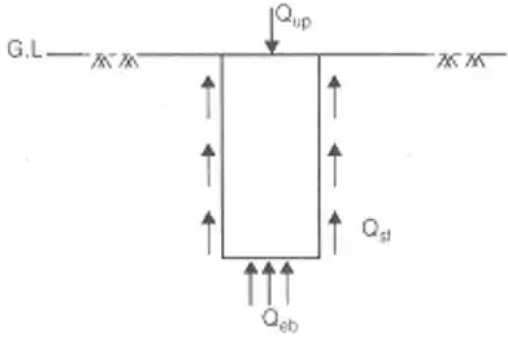(iii) qb ∼ 9C
where, C = Unit Cohesion at base of pile for clays
(iv)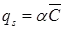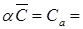Unit adhesion between pile and soil.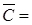Average Cohesion over depth of pile.
(v)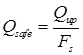where, Fs = Factor of safety.
(vi)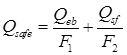F1 = 3 and F2 = 2
≈ F= F2 = 2.5
(vii) For Pure Clays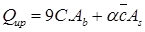2. Dynamic Approach
Dynamic methods are suitable for dense cohesionless soil only.
(i) Engineering News Records Formula
(a) Qup = WH/S + C
(b) Qap = Qup/6 = WH/(S + C)
where,
Qup = Ultimate load on pile
Qap = Allowable load on pile
W = Weight of hammer in kg.
H = Height of fall of hammer in cm.
S = Final set (Average penetration of pile per blow of hammer for last five blows in cm)
C = Constant
= 2.5 cm → for drop hammer
= 0.25 cm → for steam hammer (single acting or double acting)
(c) for drop hammer
Qap = WH/6(S + 2.5)
(d) For single Acting Stream Hammer
Qap = WH/6(S + 02.5)
(e) For Double Acting Stream Hammer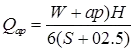where P = Stream pressure
and a = Area of hammer on which pressure acts.
(ii) Hiley Formula (I.S. Formula)where, Fs = Factor of safety = 3
ηh = Efficiency of hammer
ηb = Efficiency of blow.
ηh = 0.75 to 0.85 for single acting steam hammer
ηh = 0.75 to 0.80 for double acting steam hammer
ηh = 1 for drop hammer.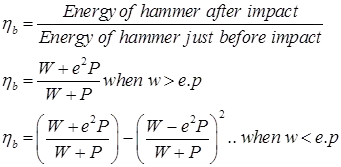where, w = Weight of hammer in kg.
p = Weight of pile + pile cap
e = Coefficient of restitutions
= 0.25 for wooden pile and cast iron hammer
= 0.4 for concrete pile and cast iron hammer
= 0.55 for steel piles and cast iron hammer
S = Final set or penetrations per blow
C = Total elastic compression of pile, pile cap and soil
H = Height of fall of hammer.
3. Field Method
(i) Use of Standard Penetrations Data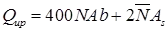where, N = Corrected S.P.T Number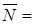Average corrected S.P.T number for entire pile length
Qap = Qup/Fs
F= Factor of safety
= 4 → For driven pile
= 2.5 → for bored pile.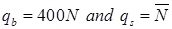(ii) Cone penetration test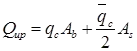where, qc = static cone resistance of the base of pile in kg/cm2
qc = average cone resistance over depth of pile in kg/cm2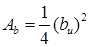Area of bulb (m)2

Under-Reamed Pile
An 'under-reamed' pile is one with an enlarged base or a bulb; the bulb is called 'under-ream'.
Under-reamed piles are cast-in-situ piles, which may be installed both in sandy and in clayey soils. The ratio of bulb size to the pile shaft size may be 2 to 3; usually a value of 2.5 is used.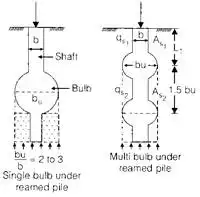As1 = πbL1qs1 = αC α < 1.
As2 = πbuL2qs2 = αC α < 1.
where, bu = dia of bulb, Spacing = 1.5 bu.
Qup = qbAb + qs1As1 + Qs2As2

Negative Skin Friction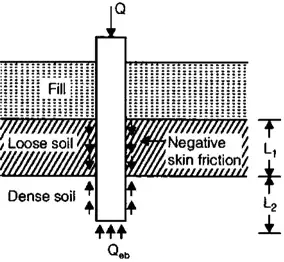1. For Cohesive sol
Qnf = Perimeter. Lα C for Cohesive soil.
where, Qnf = Total negative skin frictions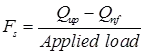where, Fs = Factor of safety.
2. For cohesionless soils
Qnf = P x force per unit surface length of pile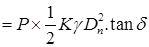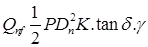(friction force = μH)
Where γ = unit weight of soil.
K = Earth pressure coefficient (Ka < K < Kp)
δ = Angle of wall friction. (φ/2 < δ < φ)

Group Action of Pile

The ultimate load carrying capacity of the pile group is finally chosen as the smaller of the

1. Ultimate load carrying capacity of n pile (n Qup)
and
2. Ultimate load carrying capacity of the single large equivalent (block) pile (Qug).

To determine design load or allowable load, apply a suitable factor of safety.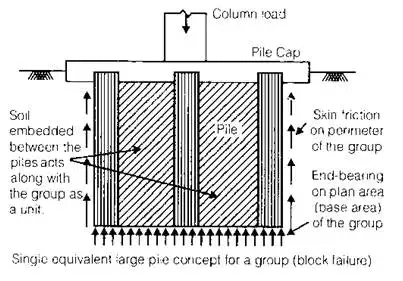1. Group Efficiency (ηg)
ng = Qug/n.Qup
Qug = Ultimate load capacity of pile group
Qup = Ultimate load on single pile
For sandy soil → ηg > 1
For clay soil → ηg < 1 and ηg > 1
Minimum number of pile for group = 3.
Qug = qbAb + qsAs
where qb = 9C for clays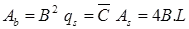(i) For Square Group
Size of group, B = (n – 1) S + D
where, η = Total number of pile if size of group is x.x
They η = x2
(ii) Qug = η.Qup
(iii) Qug = Qug/FOS where, Qug = Allowable load on pile group.
(iv) Sr = Sg/Si
where, Sr = Group settlement ratio
Sg = Settlement of pile group
Si = Settlement of individual pile.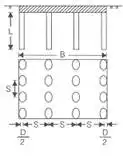2. When Piles are Embended on a Uniform Clay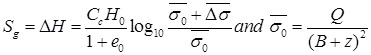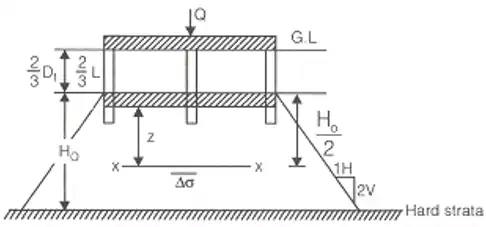3. In case of Sand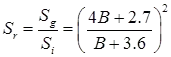where, B = Size of pile group in meter.
Offer running on EduRev: Apply code STAYHOME200 to get INR 200 off on our premium plan EduRev Infinity!

## Foundation Engineering

18 videos|20 docs|7 tests

,

,

,

,

,

,

,

,

,

,

,

,

,

,

,

,

,

,

,

,

,

;